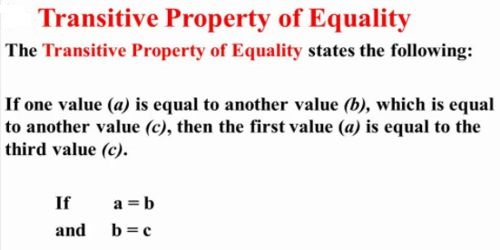Mathematic

# Transitive AxiomTransitivity is the way in which preferences are transferred logically. If product X is preferred to product Y and product Y is preferred to product Z, then it follows that product X is preferred to product Z.

Transitive Axiom: If a = b and b = c then a = c. This is the third axiom of equality. It follows Euclid’s Common Notion One: “Things equal to the same thing are equal to each other.”

It is always associated with a “greater than” or ” > ” and “less than” or ” < “. When a first element is less that the second, and the second is less than the third, therefore the third is greater than the first.

Example: when x < y, and y < z, therefore z  >  x.

The fundamental nature of algebra rests on the basic rule that whenever two numbers, variables, or expressions are equal, either one can be replaced at any time by the other one. For example, if we assume that a = b implies that b = a (the symmetric property of equality), then if I let a = 7, we have 7 = b implies b = 7. Without the basic rule, there is no way to apply the symmetric property to actual numbers.

Transitive Property – when the quantity of the first is equal to the quantity of the second and the second is equal to the quantity of the third, then the quantity of the third is equal to the quantity of the first. This property tells us that if we have two things that are equal to each other and the second thing is equal to a third thing, then the first thing is also equal to the third thing. The transitive property of equality states that if a = b and b = c, then a = c. This seems to be just a specific case of the basic rule, substituting a for b in the second assumption.

Example: z = b, b = y then y = z

or, a + 4 = b , b + 4 = a therefore  a = b

Information Source